CAT  >  Maths and Logical Reasoning Test- 3

# Maths and Logical Reasoning Test- 3

Test Description

## 15 Questions MCQ Test TISS-NET Section Wise & Full Mock Test Series | Maths and Logical Reasoning Test- 3

Maths and Logical Reasoning Test- 3 for CAT 2022 is part of TISS-NET Section Wise & Full Mock Test Series preparation. The Maths and Logical Reasoning Test- 3 questions and answers have been prepared according to the CAT exam syllabus.The Maths and Logical Reasoning Test- 3 MCQs are made for CAT 2022 Exam. Find important definitions, questions, notes, meanings, examples, exercises, MCQs and online tests for Maths and Logical Reasoning Test- 3 below.
Solutions of Maths and Logical Reasoning Test- 3 questions in English are available as part of our TISS-NET Section Wise & Full Mock Test Series for CAT & Maths and Logical Reasoning Test- 3 solutions in Hindi for TISS-NET Section Wise & Full Mock Test Series course. Download more important topics, notes, lectures and mock test series for CAT Exam by signing up for free. Attempt Maths and Logical Reasoning Test- 3 | 15 questions in 15 minutes | Mock test for CAT preparation | Free important questions MCQ to study TISS-NET Section Wise & Full Mock Test Series for CAT Exam | Download free PDF with solutions
 1 Crore+ students have signed up on EduRev. Have you?
Maths and Logical Reasoning Test- 3 - Question 1

### Direction: Study the following information and answer the question given below.M, N, P, R, T, W, F, and H are sitting around a circle facing the centre. P is third to the left of M and second to the right of T. N is second to the right of P. R is second to the right of W, who is second to the right of M. F is not an immediate neighbour of P.Who is to the immediate right of P?

Detailed Solution for Maths and Logical Reasoning Test- 3 - Question 1

According to the question, the seating arrangement of the alphabets will be as follows: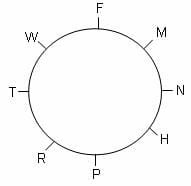H is to the immediate right of P.

Hence, the correct option is (A).

Maths and Logical Reasoning Test- 3 - Question 2

### In the following question, select the related letters from the given alternatives.ADVICE : WZREVX :: MATURE : ?

Detailed Solution for Maths and Logical Reasoning Test- 3 - Question 2

The pattern followed here is,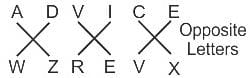Similarly,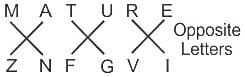Hence, the correct option is (C).

Maths and Logical Reasoning Test- 3 - Question 3

### What will come at the place of question mark ?1, 9, 25, 49, ?, 121

Detailed Solution for Maths and Logical Reasoning Test- 3 - Question 3

This question follows the following pattern:

12 = 1

32 = 9

52 = 25

72 = 49

92 = 81

112 = 121

Hence, the correct option is (D).

Maths and Logical Reasoning Test- 3 - Question 4

Direction: In the question below are given two statements followed by two conclusions numbered I and II. You have to take the given statements to be true even if they seem to be at variance with commonly known facts. Read all the conclusions and then decide which of the given conclusions logically follows from the given statements disregarding commonly known facts.

Statements:

Some pens are bags.

Some gates are bags.

Conclusions:

I. All pens are gates.

II. Some pens are gates.

Detailed Solution for Maths and Logical Reasoning Test- 3 - Question 4

The least possible Venn diagram for the given statements will be as shown: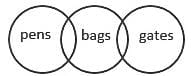Conclusions:

I. All pens are gates. → False (as there is no direct relation given between pen and gates, so this conclusion is possible but not definite)

II. Some pens are gates → False (as there is no direct relation given between pen and gates, so this conclusion is possible but not definite).

Hence, the correct option is (D).

Maths and Logical Reasoning Test- 3 - Question 5

Direction: Find the next term of the given series :

124, 62, 60, 30, 28, ?

Detailed Solution for Maths and Logical Reasoning Test- 3 - Question 5

This question follows the following pattern: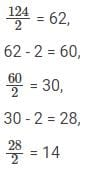Hence, the correct option is (D).

Maths and Logical Reasoning Test- 3 - Question 6

The average age of three girls is 16 years. If their ages are in the ratio 4:5:7, then the age of the youngest girl is

Detailed Solution for Maths and Logical Reasoning Test- 3 - Question 6

Let, the ages of the 3 girls be 4x years, 5x years and 7x years (ratio is 4:5:7), respectively.

Average age of the three girls = 16 years

Average age = 4x+5x+7x3

16x = 16 *3

or x = 3

Age of the youngest girl = 4x years =4×3 years

= 12years

Hence, the correct option is (C).

Maths and Logical Reasoning Test- 3 - Question 7

Directions: Study the following information to answer the given question.

Total number of passengers travelling from Patna Junction to different districts = 12000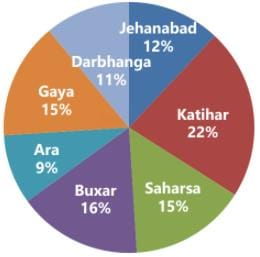The number of passengers travelling from Patna to Buxar is what per cent more than the number of passengers travelling to Jehanabad?

Detailed Solution for Maths and Logical Reasoning Test- 3 - Question 7

From the graph, The number of passengers from Patna to Buxar = 16%

Number of passengers from Patna to Jehanabad = 12%

Required percentage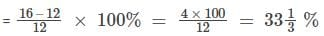Hence, the correct option is (C).

Maths and Logical Reasoning Test- 3 - Question 8

Directions: Study the following information to answer the given question.

Total number of passengers travelling from Patna Junction to different districts = 12000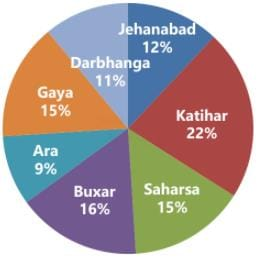What is the average number of passengers travelling from Patna to Saharsa, Katihar and Buxar together?

Detailed Solution for Maths and Logical Reasoning Test- 3 - Question 8

Total percentage of Saharsa, Katihar and Buxar = (15% + 22% + 16%) = 53%

Therefore, 53% of 12000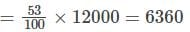Required average = 6360/3 = 2120

Hence, the correct option is (D).

Maths and Logical Reasoning Test- 3 - Question 9

Directions: Study the following information to answer the given question.

Total number of passengers travelling from Patna Junction to different districts = 12000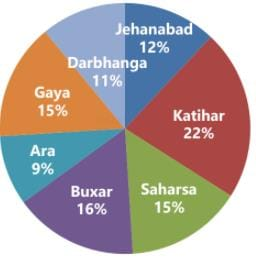The number of passengers travelling from Patna to Gaya is what per cent of the total number of passengers travelling from Patna to Darbhanga and Ara together?

Detailed Solution for Maths and Logical Reasoning Test- 3 - Question 9

Number of passengers travelling from Patna to Gaya = 15%

Number of passengers travelling from Patna to Darbhanga and Ara = 11% + 9% = 20%

Required percentage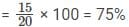Hence, the correct option is (B).

Maths and Logical Reasoning Test- 3 - Question 10

Directions: Study the following information to answer the given question.

Total number of passengers travelling from Patna Junction to different districts = 12000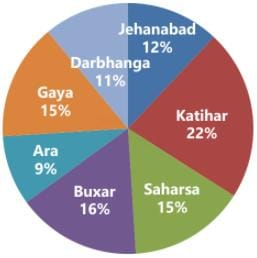Among the passengers who are travelling from Patna to Katihar, 42% are female adults and 38% are male adults. What is the number of children? (Transgenders are not to be considered.)

Detailed Solution for Maths and Logical Reasoning Test- 3 - Question 10

Number of children travelling from Patna to Katihar = 100% – (42 + 38)% = 100% – 80% = 20%

Total number of children travelling from Patna to Katihar = 20% of 22% of 12000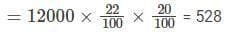Hence, the correct option is (D).

Maths and Logical Reasoning Test- 3 - Question 11

In these series, you will be looking at both the letter pattern and the number pattern. Fill the blank in the middle of the series or end of the series.

CMM, EOO, GQQ, _____, KUU

Detailed Solution for Maths and Logical Reasoning Test- 3 - Question 11

This question follows the following pattern: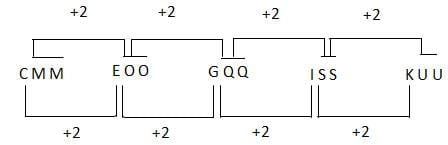Hence, the correct option is (C).

Maths and Logical Reasoning Test- 3 - Question 12

Find the odd word from the given following alternatives?

Detailed Solution for Maths and Logical Reasoning Test- 3 - Question 12

All three Tuticorin, Paradip, and Haldia are major sea ports of India except Delhi.

Hence, the correct option is (D).

Maths and Logical Reasoning Test- 3 - Question 13

A man is facing north-west. He turns 45° in the clockwise direction, then 90° in the anticlockwise direction and then another 180° in the same direction. Finally he turns 450 in clockwise direction. Which direction is he facing now?

Detailed Solution for Maths and Logical Reasoning Test- 3 - Question 13

Given,

1) A man is facing north-west.

2) He turns 45° in the clockwise direction, means towards north.

3) Then 90° in the anticlockwise direction, means towards west and then another 180° in the same direction, means towards east.

4) Finally he turns 450 in clockwise direction, means towards south - east.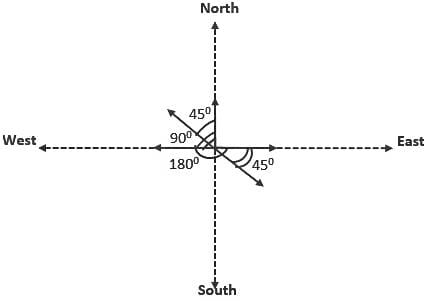Hence, the correct option is (B).

Maths and Logical Reasoning Test- 3 - Question 14

The greatest five digit number which is exactly divisible by the number 12,18,21, and 28 is:

Detailed Solution for Maths and Logical Reasoning Test- 3 - Question 14

First, we find the LCM of 12,18,21 and 28.

So, LCM of 12,18,21 and 28 is 252.

When we divide greatest five digit number 99,999 by 252, we get 207 remainder.

Now, subtracting 207 from 99,999, we get

⇒ 99,999−207=99,792

Therefore, 99,792 is largest five digit number which is divisible by 12,18,21, and 28.

Hence, the correct option is (D).

Maths and Logical Reasoning Test- 3 - Question 15

What will come at the place of question mark?

4, 7, 12, 19, 28, ?

Detailed Solution for Maths and Logical Reasoning Test- 3 - Question 15

This question follows the following pattern:

First term : 4

Second term: 4+3 = 7

Third term : 7+5 = 12

Fourth term : 12+7 = 19

Fifth term : 19+9 = 28

Therefore, Next Terms : 28+11 = 39

Hence, the correct option is (D).

## TISS-NET Section Wise & Full Mock Test Series

28 tests
 Use Code STAYHOME200 and get INR 200 additional OFF Use Coupon Code
Information about Maths and Logical Reasoning Test- 3 Page
In this test you can find the Exam questions for Maths and Logical Reasoning Test- 3 solved & explained in the simplest way possible. Besides giving Questions and answers for Maths and Logical Reasoning Test- 3, EduRev gives you an ample number of Online tests for practice

## TISS-NET Section Wise & Full Mock Test Series

28 tests

### How to Prepare for CAT

Read our guide to prepare for CAT which is created by Toppers & the best Teachers